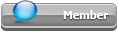## Featured Articles

Check out the latest featured articles.## File Library# Call To Arms - Some Heat Transfer Questions Of An Alumina Slab

1d unsteady state heat tra. alumina slab heat transfer

This topic has been archived. This means that you cannot reply to this topic.
5 replies to this topic
|

### #1tolgat

tolgat

Brand New Member

•• Members
•• 2 posts

Posted 12 January 2013 - 08:27 PM

my fiancee has a homework that needs to be delivered for monday and we couldn't find a way to solve them, and she refuses to meet me if she cant handle the homework, i desperately need your support for the answers,
we live in different cities and we are only able to see each other on weekends and i missed her so much, sorry for my ignorance, im an application engineer and forgot about these long time ago

Tolga

This is the question : an alumina slab with lenght of 400 mm and diameter of 130 mm , and temperature of 520 Celcius.
Is put in a room with 20 Celcius temperature, if the overall heat transfer coefficient is 50W /m2K.

after 5,15,25 minutes;

a-) what is the centerline temperature if we assume heat transfer is 1D (unsteady state, cylinder is losing temperature)
b-) what is temperature of point A,B,C,D
c-) what is total heat loss of slab### #2tolgat

tolgat

Brand New Member

•• Members
•• 2 posts

Posted 13 January 2013 - 04:36 PM

problem solved , thanks for attention

B.R.

### #3gegio1960

gegio1960

Gold Member

•• Members
•• 483 posts

Posted 15 January 2013 - 02:17 AM

Very nice.
Happy for you.... would you like to share your solution?### #4kkala

kkala

Gold Member

•• Banned
•• 1,939 posts

Posted 15 January 2013 - 01:27 PM

Perry, Section "Heat and Mass Transfer", Heat Transfer by Conduction, Unsteady-State Conduction (p 5-10 in 7th ed), gives a diagram to solve the problem for a solid cylinder of length to diameter = . Referred alumina cylinder has length / diameter = 400/130 = 3.07, yet above diagram could offer approximate indication. Using Perry's nomenclature,
t'=20 oC, tb=520 oC, rm=0.065 m, hT=50 W/m2 oC,
k=20.8 W/(m oC), c=1140 J/(kg oC), ρ=3800 kg/m3 (k, c, r from attached "alumina.pdf" at ~ 520 oC; remarkable variation, depending on grades).
parameters of Perry's diagram: m=20.8/(50*0.065)=6.4, n=65/65=1 or 0.769 or 0.154 or 0.923
1D heat transfer is understood to mean that temperature of a point does not depend on its ordinate χ (0 or 60 cm)
X = 1.136E-3θ, where θ=seconds from start of cooling; for 5, 15, 25 min X= 0.34, 1.02, 1.70 respectively.
Nevertheless Perry's diagram does not show the solution, seeing that it shows curve for m=2 and m=∞, versus m=6.4 required. Solution of relevant differential equation (cylindrical coordinates, q'=0) would be a way out. Help welcomed.

### #5breizh

breizh

Gold Member

•• ChE Plus Subscriber
•• 4,381 posts

Posted 16 January 2013 - 01:44 AM

http://wwwme.nchu.ed...r/chapter_4.pdf

You may find some interest reading this document.

Breizh

### #6kkala

kkala

Gold Member

•• Banned
•• 1,939 posts

Posted 16 January 2013 - 10:04 AM

Thanks breizh, attached article on transient heat conduction gives much more explanations than Perry. It can help application of such method, also suitable in other cases (e.g. concerning heating metal ingots).
In the referred case of alumina cylinder, it is understood (from post no 5) that Bi=1/m (m per post no 4), so Bi=1/6.4=0.16. This is close to Bi=0.1, under which temperature can be considered same in whole cylinder. Assuming so for indicative results (and using nomenclature per the article attached to post no 5), b = h*As/(ρ*V*cp) = 50*(π*0.13*0.4)/(3800*(π*0.13^2/4*0.4)*1140) = 0.0003551 /s = 0.0213 /min. Thus cylinder temperature of T oC (assumed uniform) would drop according to (T-20)/500 = exp(-0.0213*t), t in minutes; according to it T at 0, 5, 15, 25 min would be 520, 469, 383, 313 oC respectively.
One patient enough can apply the "exact solution", which does not assume uniform temperature in the cylinder. However even this cannot be precise, containing theoretical assumptions, e.g. h (heat transfer coefficient), c (heat capacity), k (thermal conductivity) independent of temperature change.